Courses
Courses for Kids
Free study material
Free LIVE classes
More

# Line And Shape## Line and Line Segment

In geometry, it is usual to suggest that a segment and a line are the same thing. A segment has a start and an endpoint, and each end is shown by a point. Segments involve the length of a table, the length of a straight road, and so on. A line, on the other hand, has no distinct beginning or endpoint. A segment is a component of a line, but a line is not a component of a segment.

## Definition of Shapes

Geometrical forms are figures that represent the shapes of various items. Some figures are two-dimensional, while others are three-dimensional. Two-dimensional figures have only the x and y axes, but three-dimensional forms have the x, y, and z axes. The z-axis represents the object's height.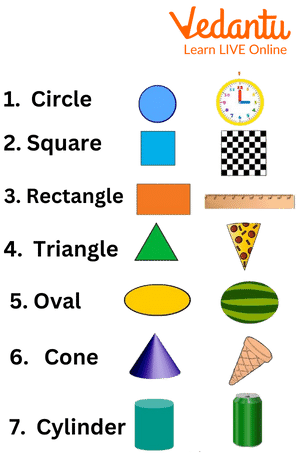Shapes Name With Example

## Define a Line

A line is a one-dimensional linear figure that stretches in opposing directions endlessly. A line is a figure produced by connecting two points with the shortest possible distance between them and extending both ends to infinity. A line can be either horizontal or vertical in orientation. It can be drawn from the left to the right or from the top to the bottom.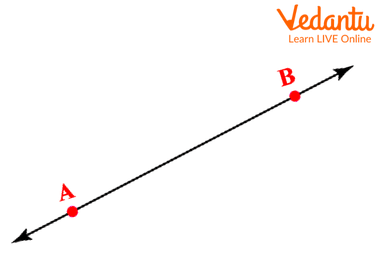Line Drawn Between Point A And B

## Types of Lines

There are different types of lines based on the operation performed on them.

Parallel lines are defined as two lines that run in the same plane but never intersect. Alternatively, if two lines do not overlap at any point, they are considered to be parallel lines.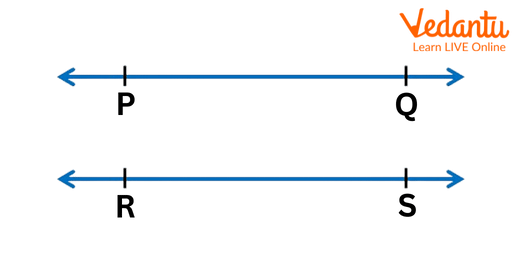Line PQ Is Parallel To Line RS

Intersecting Lines - Two lines that intersect at a place are considered to be intersecting lines. Perpendicular lines are those generated when two intersecting lines meet at a right angle. If the angle formed by two lines is a right angle, they are perpendicular to one another.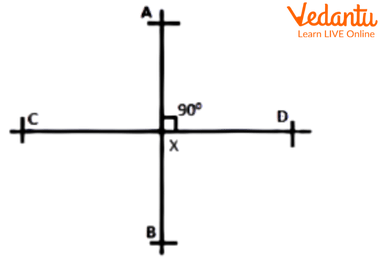Line AB Intersects Line CD at Right Angle

Transversal Line - A transversal line is one that connects two parts of lines at separate locations. A transversal line intersects two lines at points P and Q in the figure.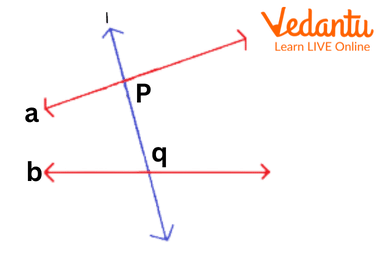Transversal Line

## Lines and Shapes Drawing

To draw any shape using lines we need to follow certain steps. Begin drawing or designing any of the figures with a line, a line segment, or a curve. Different sorts of forms and figures result from the number and arrangement of these lines, such as a triangle, a figure with three line segments joined, a pentagon (five line segments), and so on. If the lines are not joined together then the shape formed is called an open figure.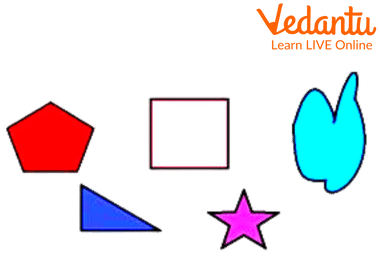Shapes Formed After Joining Different Lines

## Conclusion

A segment is a part of a line where the line can extend from one point to the other. In this unit we learnt about different types of lines and shapes. We also learnt how we can draw different shapes using the lines and line segments.

## Sample Questions

1. The given image indicates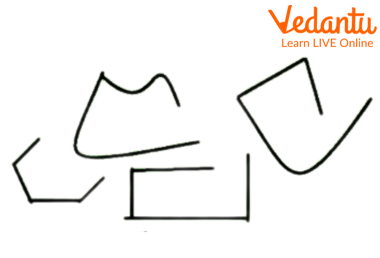Open Figures

a. Open figures

b. Closed figures

c. None of the above

d. Both a and b

Ans: Open figures

Explanation: The given image has open figures because the figures having different line segments are not joined to each other, making them incomplete figures.

2. How many angles can be formed if two lines are parallel lines?

a. Infinite

b. 360

c. 90

d. 0

Ans: 0

Explanation: Zero or no angles will be formed between the parallel lines as these lines do not intersect each other. And angles are formed only between the intersecting lines.

3. A line segment which can be extended from only one direction is called

a. Ray

b. Line segment

c. Transversal lines

d. Perpendicular line

Ans: Ray

Last updated date: 20th Sep 2023
Total views: 78.9k
Views today: 1.78k

## FAQs on Line And Shape

1. What are the shapes with no line of symmetry?

The shapes that do not have a line of symmetry are scalene triangle, parallelogram and trapezium.

2. Who invented shapes?

Euclid wrote a book named The Elements of Geometry and gave the world the invention of shapes.

3. What is the difference between horizontal and vertical lines?

The horizontal lines are the ones that are drawn horizontally or from the right to the left hand whereas the vertical lines are the ones that are drawn vertically or from the north to south direction.

4. How many lines would be required to draw an octagon?

To draw an octagon we need to have eight lines joined each other from one end to the other.

5. How many lines are required to draw a hexagon?

To draw a hexagon we need to have six lines joined each other from one end to the other.